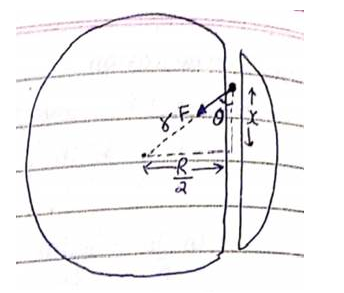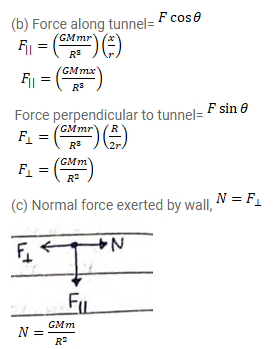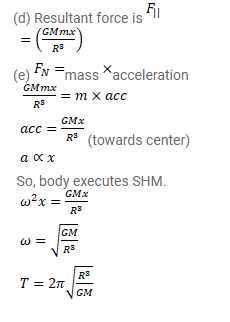# Assume that a tunnel is dug along a cord of the earth,

Question:

Assume that a tunnel is dug along a cord of the earth, at a perpendicular distance $R / 2$ from the earth's center where $R$ is the radius of the earth. The wall of the tunnel is frictionless.

(a) Find the gravitational force exerted by the earth on a particle of mass $m$ placed in the tunnel at a distance $x$ from the center of the tunnel.

(b) Find the component of this force along the tunnel and perpendicular to the tunnel.

(c) Find the normal force exerted by the wall on the particle.

(d) Find the resultant force on the particle.

(e) Show that the motion of the particle in the tunnel is simple harmonic and find the time period.

Solution: